# Detecting Muon Momentum in the CMS Experiment at CERN using Deep Learning

Posted on Thu 19 November 2020 in posts • 12 min read

# Introduction¶

In the following notebook, we will apply several Deep Learning approaches to properly predict muon momentum. We will use Monte-Carlo simulated data from the Cathode Strip Chambers (CSC) at the CMS experiment. These chambers detect muon particles in the outer layer of the CMS detector, allowing us to store information about the hit locations. The dataset contains more than 3 million muon events generated using Pythia.
You can also check the article where we summarize our project and explain the code here.

## Importing Libraries¶

Let's import the libraries that we will need in this project: First, let's import the libraries that we will need for this project:

• Numpy - for vectors manipulation and operations
• Scikit-Learn - for building and training predictive models
• Matplotlib and Seaborn - for visualizing various types of plots
• Pandas - for data cleaning and analysis
In [ ]:
import numpy as np
import sklearn
import matplotlib.pyplot as plt
import seaborn as sns
import pandas as pd

np.random.seed(48) # set the random seed for reproducible results


For training Neural Networks, we will be using Tensorflow 2 which is a famous Deep Learning library used for developing, training and deploying deep learning models.
Let's import Tensorflow and check its version:

In [ ]:
import tensorflow as tf
print(tf.__version__)

2.3.0


## Retrieving the Dataset¶

It seems our data is in NPZ format, therefore will use numpy.load() to load the data files:

In [ ]:
data=np.load("histos_tba.npz")


The data file contain two arrays: variables and parameters.

In [ ]:
data["variables"].shape,data["parameters"].shape

Out[ ]:
((3272341, 87), (3272341, 3))

We will extract the data from the variabes array for the muon hits detected at the CSC chambers only:

In [ ]:
def delete_not_csc(data):
muon_hits=data['variables']
indices_to_del=[]
for i in range(muon_hits.shape):
if(i%12>=5 and i<84):
indices_to_del.append(i)
return np.delete(muon_hits,indices_to_del,axis=1)

muon_hits=delete_not_csc(data)
muon_hits.shape

Out[ ]:
(3272341, 38)

Next, let's prepare the columns names for each feature and combine the two datasets into a Pandas DataFrame for easier analysis:

In [ ]:
original_columns_names=["Phi angle","Theta angle","Bend angle","Time",	"Ring",
columns_names=[]
for element in enumerate(original_columns_names):
for i in range(5):
columns_names.append(str(element)+str(i))

muon_hits_df=pd.DataFrame(muon_hits,columns=columns_names)
muon_hits_df["q/pt"]=data["parameters"][:,0]
muon_hits_df["Phi_angle"]=data["parameters"][:,1]
muon_hits_df["Eta_angle"]=data["parameters"][:,2]


## Exploratory Data Analysis (EDA)¶

Let's start our EDA by taking a look at the columns types:

In [ ]:
muon_hits_df.info()

<class 'pandas.core.frame.DataFrame'>
RangeIndex: 3272341 entries, 0 to 3272340
Data columns (total 41 columns):
#   Column        Dtype
---  ------        -----
0   Phi angle0    float32
1   Phi angle1    float32
2   Phi angle2    float32
3   Phi angle3    float32
4   Phi angle4    float32
5   Theta angle0  float32
6   Theta angle1  float32
7   Theta angle2  float32
8   Theta angle3  float32
9   Theta angle4  float32
10  Bend angle0   float32
11  Bend angle1   float32
12  Bend angle2   float32
13  Bend angle3   float32
14  Bend angle4   float32
15  Time0         float32
16  Time1         float32
17  Time2         float32
18  Time3         float32
19  Time4         float32
20  Ring0         float32
21  Ring1         float32
22  Ring2         float32
23  Ring3         float32
24  Ring4         float32
25  Front/Rear0   float32
26  Front/Rear1   float32
27  Front/Rear2   float32
28  Front/Rear3   float32
29  Front/Rear4   float32
38  q/pt          float32
39  Phi_angle     float32
40  Eta_angle     float32
dtypes: float32(41)
memory usage: 511.8 MB


We notice that all our variables have float data type, which we will prove helpful later on when preprocessing the data.

Next, let's generate some useful statistics about our features:

In [ ]:
muon_hits_df.describe()

Out[ ]:
Phi angle0 Phi angle1 Phi angle2 Phi angle3 Phi angle4 Theta angle0 Theta angle1 Theta angle2 Theta angle3 Theta angle4 Bend angle0 Bend angle1 Bend angle2 Bend angle3 Bend angle4 Time0 Time1 Time2 Time3 Time4 Ring0 Ring1 Ring2 Ring3 Ring4 Front/Rear0 Front/Rear1 Front/Rear2 Front/Rear3 Front/Rear4 Mask0 Mask1 Mask2 Mask3 Mask4 XRoad0 XRoad1 XRoad2 q/pt Phi_angle Eta_angle
count 2.300975e+06 870666.000000 2.789617e+06 2.605933e+06 2.439864e+06 2.300975e+06 870666.000000 2.789617e+06 2.605933e+06 2.439864e+06 2.300975e+06 870666.000000 2.789617e+06 2.605933e+06 2.439864e+06 2.300975e+06 870666.000000 2.789617e+06 2.605933e+06 2.439864e+06 2.300975e+06 870666.0 2.789617e+06 2.605933e+06 2.439864e+06 2.300975e+06 870666.000000 2.789617e+06 2.605933e+06 2.439864e+06 3.272341e+06 3.272341e+06 3.272341e+06 3.272341e+06 3.272341e+06 3.272341e+06 3.272341e+06 3.272341e+06 3.272341e+06 3.272341e+06 3.272341e+06
mean 2.898916e+03 2942.738281 2.884543e+03 2.895544e+03 2.901002e+03 2.513442e+01 62.888813 3.345796e+01 3.376106e+01 3.479657e+01 1.771088e-02 -0.075262 2.404990e-03 7.820615e-04 1.634517e-03 -1.554471e-02 -0.029150 -2.238408e-02 -2.388741e-02 -1.674315e-02 2.208553e+00 2.0 1.234539e+00 1.328101e+00 1.416067e+00 4.993205e-01 0.465420 5.033727e-01 4.964771e-01 5.009226e-01 2.968413e-01 7.339318e-01 1.475164e-01 2.036487e-01 2.543980e-01 3.977467e+00 2.312063e+00 9.032854e+01 -1.427713e-04 -4.566138e-04 -1.287372e-03
std 1.063501e+03 1061.839233 1.087773e+03 1.087355e+03 1.084414e+03 1.279694e+01 10.498766 2.147677e+01 2.178618e+01 2.156608e+01 1.678599e+01 19.846676 3.044079e-01 3.709632e-01 3.650869e-01 1.228342e-01 0.167572 1.469481e-01 1.515253e-01 1.274973e-01 1.475620e+00 0.0 4.290860e-01 4.634236e-01 4.966435e-01 5.000001e-01 0.499028 4.999973e-01 4.999975e-01 5.000001e-01 4.557488e-01 4.387615e-01 3.566231e-01 3.976815e-01 4.353520e-01 1.956127e+00 1.881727e+00 3.388365e+01 2.625431e-01 1.811694e+00 1.889245e+00
min 6.620000e+02 690.000000 9.600000e+01 9.400000e+01 9.600000e+01 5.000000e+00 46.000000 5.000000e+00 5.000000e+00 6.000000e+00 -1.329103e+02 -81.000000 -1.000000e+00 -1.000000e+00 -1.000000e+00 -1.000000e+00 -1.000000 -1.000000e+00 -1.000000e+00 -1.000000e+00 1.000000e+00 2.0 1.000000e+00 1.000000e+00 1.000000e+00 0.000000e+00 0.000000 0.000000e+00 0.000000e+00 0.000000e+00 0.000000e+00 0.000000e+00 0.000000e+00 0.000000e+00 0.000000e+00 0.000000e+00 0.000000e+00 2.000000e+00 -4.999985e-01 -3.141591e+00 -2.499856e+00
25% 2.005000e+03 2032.000000 1.980000e+03 1.998000e+03 2.000000e+03 1.400000e+01 54.000000 1.600000e+01 1.600000e+01 1.600000e+01 -1.200000e+01 -14.000000 0.000000e+00 0.000000e+00 0.000000e+00 0.000000e+00 0.000000 0.000000e+00 0.000000e+00 0.000000e+00 1.000000e+00 2.0 1.000000e+00 1.000000e+00 1.000000e+00 0.000000e+00 0.000000 0.000000e+00 0.000000e+00 0.000000e+00 0.000000e+00 0.000000e+00 0.000000e+00 0.000000e+00 0.000000e+00 3.000000e+00 1.000000e+00 6.200000e+01 -2.194096e-01 -1.571401e+00 -1.880253e+00
50% 2.891000e+03 2937.000000 2.882000e+03 2.896000e+03 2.904000e+03 2.300000e+01 62.000000 2.800000e+01 2.800000e+01 2.900000e+01 0.000000e+00 0.000000 0.000000e+00 0.000000e+00 0.000000e+00 0.000000e+00 0.000000 0.000000e+00 0.000000e+00 0.000000e+00 1.000000e+00 2.0 1.000000e+00 1.000000e+00 1.000000e+00 0.000000e+00 0.000000 1.000000e+00 0.000000e+00 1.000000e+00 0.000000e+00 1.000000e+00 0.000000e+00 0.000000e+00 0.000000e+00 4.000000e+00 2.000000e+00 9.000000e+01 -4.459424e-04 7.330791e-04 -1.202088e+00
75% 3.792000e+03 3853.000000 3.791000e+03 3.795000e+03 3.800000e+03 3.500000e+01 71.000000 4.700000e+01 5.200000e+01 5.200000e+01 1.200000e+01 14.000000 0.000000e+00 0.000000e+00 0.000000e+00 0.000000e+00 0.000000 0.000000e+00 0.000000e+00 0.000000e+00 4.000000e+00 2.0 1.000000e+00 2.000000e+00 2.000000e+00 1.000000e+00 1.000000 1.000000e+00 1.000000e+00 1.000000e+00 1.000000e+00 1.000000e+00 0.000000e+00 0.000000e+00 1.000000e+00 5.000000e+00 4.000000e+00 1.190000e+02 2.191571e-01 1.569422e+00 1.879843e+00
max 4.987000e+03 4950.000000 4.954000e+03 4.952000e+03 4.952000e+03 5.300000e+01 88.000000 8.800000e+01 8.800000e+01 8.800000e+01 1.421401e+02 70.000000 1.000000e+00 1.000000e+00 1.000000e+00 0.000000e+00 0.000000 0.000000e+00 0.000000e+00 0.000000e+00 4.000000e+00 2.0 2.000000e+00 2.000000e+00 2.000000e+00 1.000000e+00 1.000000 1.000000e+00 1.000000e+00 1.000000e+00 1.000000e+00 1.000000e+00 1.000000e+00 1.000000e+00 1.000000e+00 8.000000e+00 5.000000e+00 1.540000e+02 4.999996e-01 3.141592e+00 2.499996e+00

We notice that some features such as Phi angle1, Theta angle1 and Bend angle1 have some missing values.
Let's find the exact percentage of null values for each feature:

In [ ]:
null_perc=((muon_hits_df.isnull().
sum()/muon_hits_df.
shape
)*100).sort_values(ascending=False)

fig = plt.figure(figsize = (10,5))
ax = fig.gca()
ax.set_xlabel("features names")
ax.set_ylabel("missing values percentage")

null_perc.plot.bar(ax=ax)
plt.show()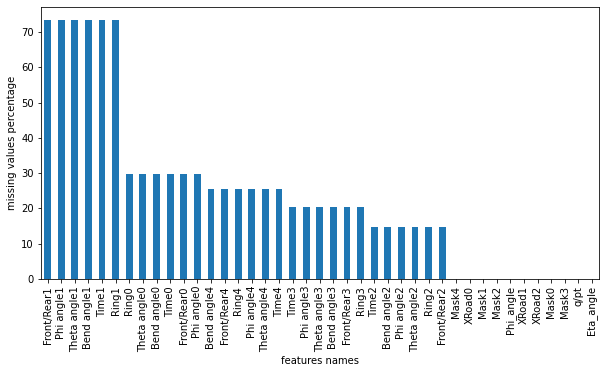We notice that Front/Rear1, Phi angle1, Theta angle1, Bend angle1, Time1 and Ring1 features have more than 70% missing values!
Next, let's take a look on the data distribution of each feature:

In [ ]:
fig = plt.figure(figsize = (30,40))
ax = fig.gca()
muon_hits_df.hist(ax=ax)
plt.show()

/usr/local/lib/python3.6/dist-packages/ipykernel_launcher.py:3: UserWarning: To output multiple subplots, the figure containing the passed axes is being cleared
This is separate from the ipykernel package so we can avoid doing imports until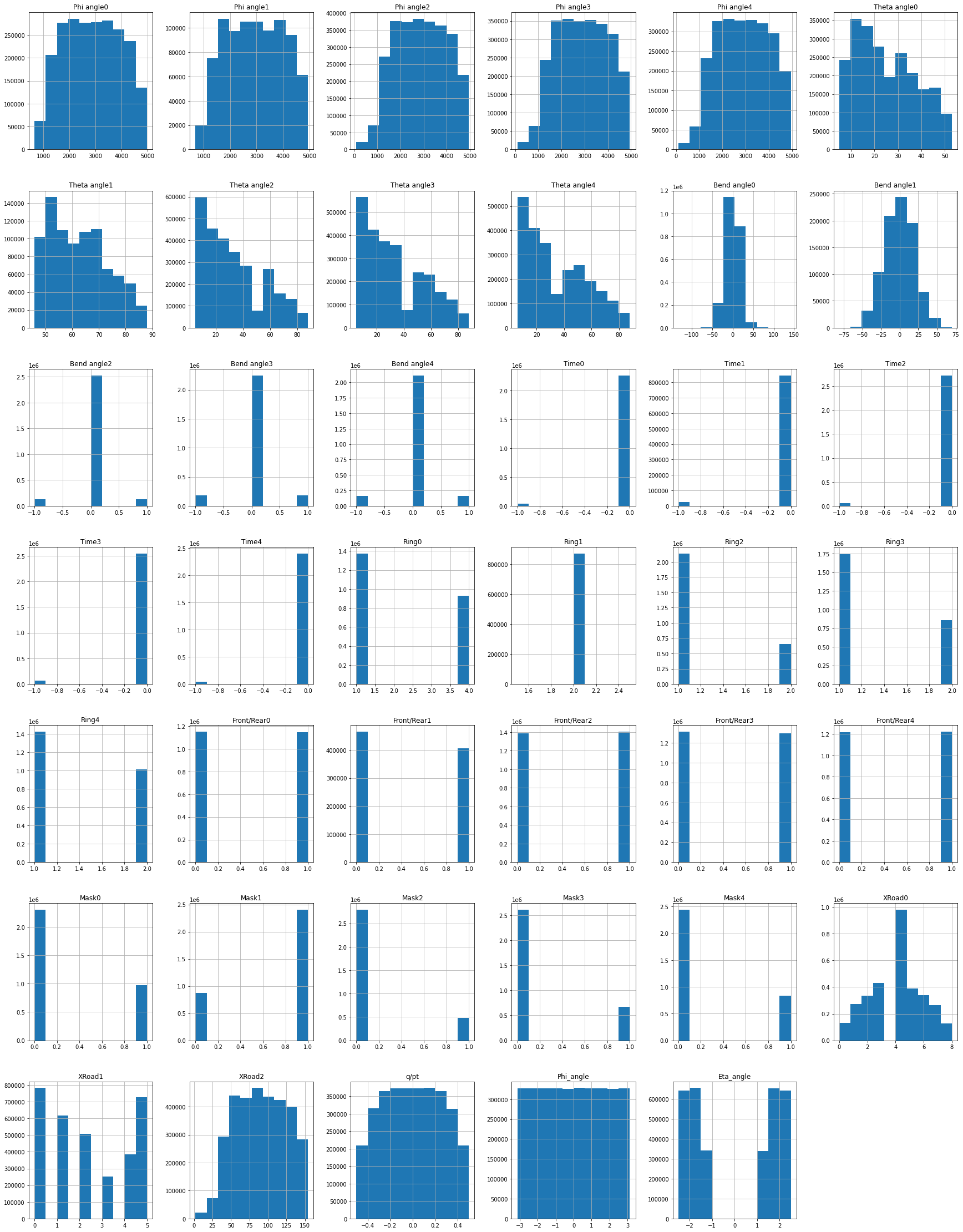Wow, those are a LOT of plots! It is clear from these plots that some features such as Theta Angle1,Theta Angle2, Theta Angle3 and XRoad1 can benefit from being transformed into Normal Distribution (Standardization).

Next, let's investigate the effect of each feature on the target feature q/pt:

In [ ]:
corr=muon_hits_df.corr()
f, ax = plt.subplots(figsize=(11, 9))
cmap = sns.diverging_palette(230, 20, as_cmap=True)
square=True, linewidths=.5, cbar_kws={"shrink": .5})

Out[ ]:
<matplotlib.axes._subplots.AxesSubplot at 0x7f6a2cb0de48>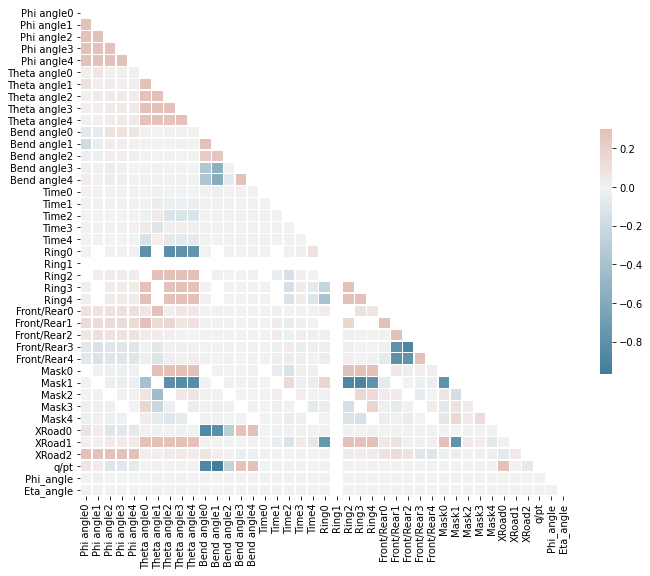# Framing the Problem as a Classification Task¶

Instead of trying to apply regression to predict the muon momenta values (q/pt), let's try to cluster the momenta into 4 classes: 0-10 GeV, 10-30 GeV, 30-100 GeV and >100 GeV, and instead frame the problem as a classification task.

Let's investigate the q/pt column and separate it from the data:

In [ ]:
pt_inverse_labels=muon_hits_df["q/pt"]
muon_hits_df_x=muon_hits_df.drop("q/pt",axis=1)
muon_hits_df["q/pt"].describe()

Out[ ]:
count    3.272341e+06
mean    -1.427713e-04
std      2.625431e-01
min     -4.999985e-01
25%     -2.194096e-01
50%     -4.459424e-04
75%      2.191571e-01
max      4.999996e-01
Name: q/pt, dtype: float64

We will use the absolute value of the reciprocal of the target feature (pt/q) to group the momenta into the previously mentioned classes:

In [ ]:
pt_labels=abs((1/pt_inverse_labels))
pt_labels.describe()

Out[ ]:
count    3.272341e+06
mean     1.859705e+01
std      1.257423e+02
min      2.000001e+00
25%      2.998728e+00
50%      4.560210e+00
75%      9.115835e+00
max      6.989345e+03
Name: q/pt, dtype: float64

Let's visualize the data distribution of this feature:

In [ ]:
pt_labels.hist(bins=1000)
plt.xlim([0,150])
plt.title("Data Distribution of p_T labels")
plt.show()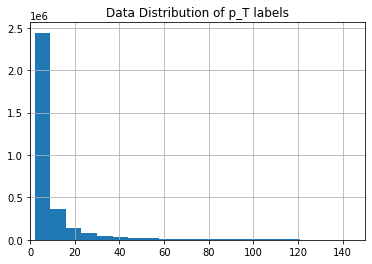That is a very interesting plot! We can clearly see that there are distinct groups of points of different magnitudes.
We can now group the values into 4 groups: 0-10 GeV, 10-30 GeV, 30-100 GeV and >100 GeV using the cut method from Pandas.

In [ ]:
bins = pd.IntervalIndex.from_tuples([(0, 10), (10, 30), (30, 100),(100,pt_labels.max()+1)])
org_pt_labels_groups=pd.cut(pt_labels, bins)


Let's plot the grouped data now:

In [ ]:
target_counts=org_pt_labels_groups.value_counts()
target_counts.plot.bar()
plt.title("Distribution of classes in target feature")
plt.show()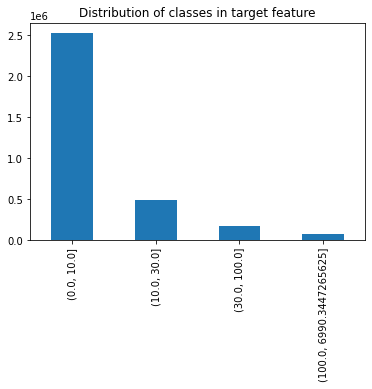It is very clear that the classes are not balanced, therefore it is important to balance those classes whenever we start training our Neural Network to avoid any bias.

### Splitting the Data¶

Next, let's seperate the data into train and test data, we will use a 90%-10% split.

As we already noticed, the data is highly imbalanced, therefore it is important to perform the following two tasks:

• Add class weights to balance out the classes
• Make the test set representative of the classes

To split the train and test data, we will use the StratifiedShuffleSplit method in Scikit-Learn to make both the train data and test data representative of the target classes distribution:

In [ ]:
from sklearn.model_selection import StratifiedShuffleSplit

split = StratifiedShuffleSplit(n_splits=1, test_size=0.1, random_state=42)
for train_index, test_index in split.split(muon_hits_df_x, org_pt_labels_groups):
muon_hits_x_train_set = muon_hits_df_x.loc[train_index]
pt_labels_groups_y_train_set=org_pt_labels_groups[train_index]
muon_hits_x_test_set = muon_hits_df_x.loc[test_index]
pt_labels_groups_y_test_set=org_pt_labels_groups[test_index]


Let's verify if the data has the same proportions for the target label in the train and test sets:

In [ ]:
def target_proportions(data):
return data.value_counts()/len(data)*100

proportions_df=pd.DataFrame({"Original": target_proportions(org_pt_labels_groups),
"Train":target_proportions(pt_labels_groups_y_train_set),
"Test":target_proportions(pt_labels_groups_y_test_set)},
)

proportions_df

Out[ ]:
Original Train Test
(0.0, 10.0] 77.201062 77.201058 77.201094
(10.0, 30.0] 15.207951 15.207942 15.208031
(30.0, 100.0] 5.331871 5.331862 5.331948
(100.0, 6990.3447265625] 2.259117 2.259138 2.258927

Neat! Now that we are sure that the train and test data are representative of the original dataset, we can now proceed to the preprocessing phase.

### Preparing data for training¶

As we noticed earlier, we need delete the columns with percentage of missing values bigger than 70%:

In [ ]:
def delete_columns(df,perc):
null_perc=(df.isnull().sum()/df.shape)*100
col_to_del= [col for col in df.columns if(((null_perc)>perc)[col])]
print("columns deleted:", col_to_del)
return df.drop(col_to_del,axis=1)

muon_hits_x_train_set_1=delete_columns(muon_hits_x_train_set,70)

columns deleted: ['Phi angle1', 'Theta angle1', 'Bend angle1', 'Time1', 'Ring1', 'Front/Rear1']

In [ ]:
muon_hits_x_train_set_1.shape

Out[ ]:
(2945106, 34)

For all other features with percentage of null values less than 70%, we will replace the null values with the mean value of their corresponding columns:

In [ ]:
def replace_missing_with_mean(df):
from sklearn.impute import SimpleImputer
imputer = SimpleImputer(missing_values=np.nan, strategy='mean')
imputed=imputer.fit_transform(df)
return pd.DataFrame(imputed,columns=df.columns)

In [ ]:
muon_hits_x_train_set_2=replace_missing_with_mean(muon_hits_x_train_set_1)

In [ ]:
null_perc_2=(muon_hits_x_train_set_2.isnull().
sum()/muon_hits_x_train_set_2.
shape)*100
null_perc_2.sort_values(ascending=False)

Out[ ]:
Eta_angle       0.0
Bend angle0     0.0
Time3           0.0
Time2           0.0
Time0           0.0
Bend angle4     0.0
Bend angle3     0.0
Bend angle2     0.0
Theta angle4    0.0
Phi_angle       0.0
Theta angle3    0.0
Theta angle2    0.0
Theta angle0    0.0
Phi angle4      0.0
Phi angle3      0.0
Phi angle2      0.0
Time4           0.0
Ring0           0.0
Ring2           0.0
Ring3           0.0
Ring4           0.0
Front/Rear0     0.0
Front/Rear2     0.0
Front/Rear3     0.0
Front/Rear4     0.0
Phi angle0      0.0
dtype: float64

Now we are sure that we filled all missing values.

Let's next create the preprocessing pipeline and convert the target feature into one hot encoded variables.

In [ ]:
def preprocess_pipeline(df_x,df_y):
#delete columns with missing values more than 70%
df_x=delete_columns(df_x,70)

#impute the other columns with missing values with their corresponding means
df_x=replace_missing_with_mean(df_x)

#standardize the data
from sklearn.preprocessing import StandardScaler
scaler=StandardScaler()
x=scaler.fit_transform(df_x)

#convert labels into dummy variables (one hot encoded)
from sklearn.preprocessing import LabelEncoder
encoder = LabelEncoder()
encoded_y = encoder.fit_transform(df_y)
print(encoder.classes_)

from sklearn.preprocessing import OneHotEncoder
ohe=OneHotEncoder(sparse=False)
ohe_y=ohe.fit_transform(encoded_y.reshape(-1,1))
print(ohe.categories_)

return x,ohe_y,encoded_y

In [ ]:
muon_hits_x_train_values,pt_labels_ohe_y_train,y_encoded_train=preprocess_pipeline(muon_hits_x_train_set,pt_labels_groups_y_train_set)

columns deleted: ['Phi angle1', 'Theta angle1', 'Bend angle1', 'Time1', 'Ring1', 'Front/Rear1']
[Interval(0.0, 10.0, closed='right') Interval(10.0, 30.0, closed='right')
Interval(30.0, 100.0, closed='right')
Interval(100.0, 6990.3447265625, closed='right')]
[array([0, 1, 2, 3])]

In [ ]:
muon_hits_x_test_values,pt_labels_ohe_y_test,y_encoded_test=preprocess_pipeline(muon_hits_x_test_set,pt_labels_groups_y_test_set)

columns deleted: ['Phi angle1', 'Theta angle1', 'Bend angle1', 'Time1', 'Ring1', 'Front/Rear1']
[Interval(0.0, 10.0, closed='right') Interval(10.0, 30.0, closed='right')
Interval(30.0, 100.0, closed='right')
Interval(100.0, 6990.3447265625, closed='right')]
[array([0, 1, 2, 3])]


Now our datasets are ready for training and testing!
One last task to do before training, which is generating the classes weights:

In [ ]:
#option 1
from sklearn.utils import class_weight

class_weights = class_weight.compute_class_weight('balanced',
np.unique(y_encoded_train),
y_encoded_train)

In [ ]:
class_weights

Out[ ]:
array([ 0.32382976,  1.64387796,  4.68879315, 11.06616918])
In [ ]:
#option 2
class_weights=len(y_encoded_train)/(4*(np.unique(y_encoded_train,return_counts=True)))
classes_weights={i: class_weights[i] for i in range(len(class_weights))}
classes_weights

Out[ ]:
{0: 0.3238297576631087,
1: 1.6438779611065217,
2: 4.688793152857116,
3: 11.066169176661557}

### Building the Neural Network¶

We will use Tensorflow's Keras library to build the Neural Network

### Building a Fully Connected Neural Network¶

Let's first build and compile the model:

In [ ]:
from tensorflow.keras import Sequential
from tensorflow.keras.layers import Dense,Dropout
model=Sequential()



Let's take a closer look at the model architecture:

In [ ]:
model.summary()

Model: "sequential"
_________________________________________________________________
Layer (type)                 Output Shape              Param #
=================================================================
dense (Dense)                (None, 512)               17920
_________________________________________________________________
dense_1 (Dense)              (None, 256)               131328
_________________________________________________________________
dropout (Dropout)            (None, 256)               0
_________________________________________________________________
dense_2 (Dense)              (None, 128)               32896
_________________________________________________________________
dense_3 (Dense)              (None, 64)                8256
_________________________________________________________________
dropout_1 (Dropout)          (None, 64)                0
_________________________________________________________________
dense_4 (Dense)              (None, 32)                2080
_________________________________________________________________
dropout_2 (Dropout)          (None, 32)                0
_________________________________________________________________
dense_5 (Dense)              (None, 4)                 132
=================================================================
Total params: 192,612
Trainable params: 192,612
Non-trainable params: 0
_________________________________________________________________


Next, we will set some callbacks for the model including a Checkpoint callback and Early Stopping callback that both monitor the model's validation accuracy:

In [ ]:
from tensorflow.keras.callbacks import ModelCheckpoint
from tensorflow.keras.callbacks import EarlyStopping

filepath="classifier_weights2-improvement-{epoch:02d}-{val_accuracy:.2f}.hdf5"
checkpoint1 = ModelCheckpoint(filepath, monitor='val_accuracy', verbose=1, save_best_only=True, mode='max')
checkpoint2= EarlyStopping( monitor='val_accuracy', patience=3)

callbacks_list = [checkpoint1,checkpoint1]


Now we can finally start training!
We will use a 25% validation split, batch size of 500 and will train for 100 epochs.

In [ ]:
history = model.fit(muon_hits_x_train_values,  pt_labels_ohe_y_train,
validation_split=0.25,
epochs=200,
batch_size=500,
callbacks=callbacks_list,
class_weight=classes_weights)


Next, let's plot the evolution of the metrics over the epochs of training:

In [ ]:
# list all data in history
print(history.history.keys())

# summarize history for accuracy
plt.plot(history.history['accuracy'])
plt.plot(history.history['val_accuracy'])
plt.title('model accuracy')
plt.ylabel('accuracy')
plt.xlabel('epoch')
plt.legend(['train', 'test'], loc='upper left')
plt.savefig("accuracy2.png")
plt.show()

# summarize history for loss
plt.plot(history.history['loss'])
plt.title('model train loss')
plt.ylabel('loss')
plt.xlabel('epoch')
plt.savefig("loss2-1.png")
plt.show()

plt.plot(history.history['val_loss'])
plt.title('model validation loss')
plt.ylabel('loss')
plt.xlabel('epoch')
plt.savefig("loss2-2.png")
plt.show()

dict_keys(['loss', 'accuracy', 'val_loss', 'val_accuracy'])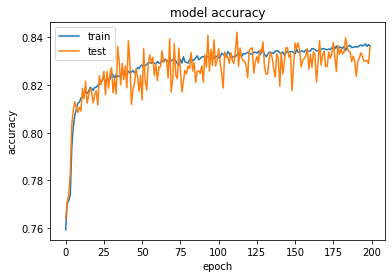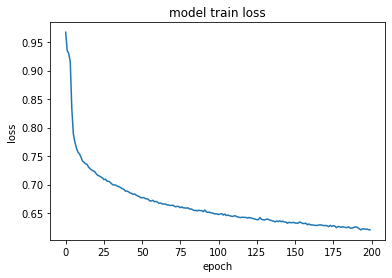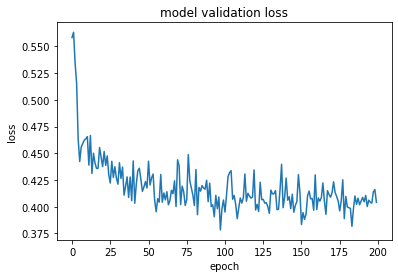Let's load the model with the best performance:

In [ ]:
model.load_weights("/content/classifier_weights-improvement-94-0.84.hdf5")


### Testing the Model¶

We will examine the accuracy of the model on the train set and the test set:
Let's generate a classification report that will give us insights on the performance of the model on both train set and test set:

In [ ]:
from sklearn.metrics import classification_report
y_train_pred=model.predict(muon_hits_x_train_values)
y_train_pred_best=np.argmax(y_train_pred,axis=1)
train_report=classification_report(y_encoded_train,y_train_pred_best)

In [ ]:
y_test_pred=model.predict(muon_hits_x_test_values)
y_test_pred_best=np.argmax(y_test_pred,axis=1)
test_report=classification_report(y_encoded_test,y_test_pred_best)

In [ ]:
print("Train report \n",train_report)
print("Test report \n",test_report)

Train report
precision    recall  f1-score   support

0       0.99      0.90      0.94   2273653
1       0.65      0.59      0.62    447890
2       0.33      0.73      0.46    157029
3       0.36      0.66      0.47     66534

accuracy                           0.84   2945106
macro avg       0.58      0.72      0.62   2945106
weighted avg       0.89      0.84      0.86   2945106

Test report
precision    recall  f1-score   support

0       0.99      0.90      0.94    252629
1       0.64      0.58      0.61     49766
2       0.32      0.70      0.44     17448
3       0.33      0.61      0.43      7392

accuracy                           0.84    327235
macro avg       0.57      0.70      0.61    327235
weighted avg       0.89      0.84      0.85    327235



That's impressive! It seems that adding class weights definitely reduced bias and we reached a weighted f1-score of 0.85 on the test data!

# Experimenting with Regression¶

Instead of classifying into four group, let us try to directly predict the q/pT value for each muon momentum:

## Splitting the Data¶

Let us first split the dataset into train and test data:

In [ ]:
from sklearn.model_selection import train_test_split
muon_hits_x_train,muon_hits_x_test,pt_inverse_labels_train, pt_inverse_labels_test = train_test_split(muon_hits_df_x,pt_inverse_labels,test_size=0.1, random_state=42)


Next, let's create the pipeline for preprocessing the data for regression:

In [ ]:
def preprocess_pipeline_reg(df_x,df_y):
#delete columns with missing values more than 70%
df_x=delete_columns(df_x,70)

#impute the other columns with missing values with their corresponding means
df_x=replace_missing_with_mean(df_x)

#standardize the data
from sklearn.preprocessing import StandardScaler
scaler=StandardScaler()
x=scaler.fit_transform(df_x)

return x,df_y.values

In [ ]:
muon_hits_x_train_values,pt_inverse_labels_train_values=preprocess_pipeline_reg(muon_hits_x_train, pt_inverse_labels_train)
muon_hits_x_test_values,pt_inverse_labels_test_values=preprocess_pipeline_reg(muon_hits_x_test, pt_inverse_labels_test)

columns deleted: ['Phi angle1', 'Theta angle1', 'Bend angle1', 'Time1', 'Ring1', 'Front/Rear1']
columns deleted: ['Phi angle1', 'Theta angle1', 'Bend angle1', 'Time1', 'Ring1', 'Front/Rear1']


## Building the Model¶

Let's first build and compile the model:

In [ ]:
from tensorflow.keras import Sequential
from tensorflow.keras.layers import Dense,Dropout
reg_model=Sequential()


In [ ]:
reg_model.summary()

Model: "sequential"
_________________________________________________________________
Layer (type)                 Output Shape              Param #
=================================================================
dense (Dense)                (None, 512)               17920
_________________________________________________________________
dense_1 (Dense)              (None, 256)               131328
_________________________________________________________________
dropout (Dropout)            (None, 256)               0
_________________________________________________________________
dense_2 (Dense)              (None, 128)               32896
_________________________________________________________________
dense_3 (Dense)              (None, 64)                8256
_________________________________________________________________
dropout_1 (Dropout)          (None, 64)                0
_________________________________________________________________
dense_4 (Dense)              (None, 32)                2080
_________________________________________________________________
dropout_2 (Dropout)          (None, 32)                0
_________________________________________________________________
dense_5 (Dense)              (None, 1)                 33
=================================================================
Total params: 192,513
Trainable params: 192,513
Non-trainable params: 0
_________________________________________________________________


Next, we will set some callbacks for the model including a Checkpoint callback and Early Stopping callback that will both monitor the model's validation mean square error:

In [ ]:
from tensorflow.keras.callbacks import ModelCheckpoint
from tensorflow.keras.callbacks import EarlyStopping

filepath="reg_weights2-improvement-{epoch:02d}-{val_loss:.6f}.hdf5"
checkpoint1 = ModelCheckpoint(filepath, monitor='val_loss', verbose=1, save_best_only=True, mode='min')
checkpoint2= EarlyStopping( monitor='val_loss', patience=3)

callbacks_list = [checkpoint1,checkpoint1]

In [ ]:
reg_history = reg_model.fit(muon_hits_x_train_values, pt_inverse_labels_train_values, validation_split=0.25, epochs=200, batch_size=2000,callbacks=callbacks_list)


Next, let's plot the evolution of the model over the epochs of training:

In [ ]:
# list all data in history
print(reg_history.history.keys())

# summarize history for loss
plt.plot(reg_history.history['loss'])
plt.plot(reg_history.history['val_loss'])
plt.title('model loss')
plt.ylabel('loss')
plt.xlabel('epoch')
plt.legend(['train', 'test'], loc='upper left')
plt.savefig("reg_loss.png")
plt.show()

dict_keys(['loss', 'val_loss'])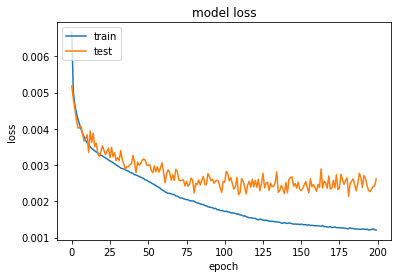## Model Testing¶

Let's evaluate the model performance over the train and test set:

In [ ]:
reg_model.load_weights("/content/reg_weights2-improvement-81-0.002230.hdf5")

In [ ]:
from sklearn.metrics import mean_squared_error
y_train_pred_reg=reg_model.predict(muon_hits_x_train_values)
print("train root mean squared error: ",mean_squared_error(pt_inverse_labels_train_values,y_train_pred_reg,squared=False))

y_test_pred_reg=reg_model.predict(muon_hits_x_test_values)
print("test root mean squared error: ",mean_squared_error(pt_inverse_labels_test_values,y_test_pred_reg,squared=False))

train root mean squared error:  0.046178654
test root mean squared error:  0.047247976


That is impressive! the root mean square error on the testing data is relatively small in comparison to the data!

# Future Directions¶

• We discovered in this project that the classifier model was not very good in detecting minority classes. Therefore we need to look for more techniques to make the model less biased (such as up sampling minority classes or down sampling the majority class).
• We can further improve the performance of our regression model by hyperparameters tuning through grid search.